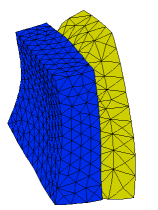﻿ Sample Problems > Applications > Stress > 3d_bimetal

# 3d_bimetal

Navigation:  Sample Problems > Applications > Stress >

# 3d_bimetal{ 3D_BIMETAL.PDE

This problem considers a small block of aluminum bonded to a larger block

of iron.  The assembly is held at a fixed temperature at the bottom, and

is convectively cooled on the sides and top.  We solve for the 3D temperature

distribution, and the associated deformation and stress.

All faces of the assembly are unconstrained, allowing it to grow as the

temperature distribution demands.  We do not use an integral constraint

to cancel translation and rotation, as we have done in 2D samples,

because in 3D this is very expensive.  Instead, we let FlexPDE find a solution,

and then remove the mean translation and rotation before plotting.

 }   title 'Bimetal Part'   coordinates    cartesian3   select    painted   { show color-filled contours }    biprecon { use the Block-Inverse preconditioner - it works better than the default ICCG }   variables    Tp       { temperature difference from stress-free state }    U         { X displacement }    V         { Y displacement }    W         { Z displacement }   materials   'iron' :       K = 0.11           { thermal conductivity }       E = 20e11         { Youngs modulus }       nu = 0.28         { expansion coefficient }       alpha =  1.7e-6   { Poisson's Ratio  }     'aluminum' :       K = 0.5       E = 6e11       nu = 0.25       alpha =  2*(2.6e-6)             ! Exaggerate expansion     'default' :       K = 1       E = 1e11       nu = 0.1       alpha =  1e-6   definitions    long = 1    wide = 0.3    high = 1    tabx = 0.2    taby = 0.4Q = 0               { Thermal source }

Ta = 0.             { define the ambient thermal sink temperature }

{ define the constitutive relations }

G = E/((1+nu)*(1-2*nu))

C11 = G*(1-nu)

C12 = G*nu

C13 = G*nu

C22 = G*(1-nu)

C23 = G*nu

C33 = G*(1-nu)

C44 = G*(1-2*nu)/2

b = G*alpha*(1+nu)

{ Strains }

ex = dx(U)

ey = dy(V)

ez = dz(W)

gxy = dy(U) + dx(V)

gyz = dz(V) + dy(W)

gzx = dx(W) + dz(U)

{ Stresses }

Sx  =  C11*ex + C12*ey + C13*ez - b*Tp

Sy  =  C12*ex + C22*ey + C23*ez - b*Tp

Sz  =  C13*ex + C23*ey + C33*ez - b*Tp

Txy =  C44*gxy

Tyz =  C44*gyz

Tzx =  C44*gzx

{ find mean translation and rotation }

Vol = Integral(1)

Tx = integral(U)/Vol                   { X-motion }

Ty = integral(V)/Vol                   { Y-motion }

Tz = integral(W)/Vol                   { Z-motion }

Rz = 0.5*integral(dx(V) - dy(U))/Vol   { Z-rotation }

Rx = 0.5*integral(dy(W) - dz(V))/Vol   { X-rotation }

Ry = 0.5*integral(dz(U) - dx(W))/Vol   { Y-rotation }

{ displacements with translation and rotation removed }

{ This is necessary only if all boundaries are free }

Up = U - Tx + Rz*y - Ry*z

Vp = V - Ty + Rx*z - Rz*x

Wp = W - Tz + Ry*x - Rx*y

{ scaling factors for displacement plots }

Mx = 0.2*globalmax(magnitude(y,z))/globalmax(magnitude(Vp,Wp))

My = 0.2*globalmax(magnitude(x,z))/globalmax(magnitude(Up,Wp))

Mz = 0.2*globalmax(magnitude(x,y))/globalmax(magnitude(Up,Vp))

Mt = 0.4*globalmax(magnitude(x,y,z))/globalmax(magnitude(Up,Vp,Wp))

initial values

Tp = 5.

U = 1.e-5

V = 1.e-5

W = 1.e-5

equations

Tp: div(k*grad(Tp)) + Q = 0.         { the heat equation }

U:  dx(Sx) + dy(Txy) + dz(Tzx) = 0   { the U-displacement equation }

V:  dx(Txy) + dy(Sy) + dz(Tyz) = 0   { the V-displacement equation }

W:  dx(Tzx) + dy(Tyz) + dz(Sz) = 0   { the W-displacement equation }

extrusion z = 0,long

boundaries

surface 1 value(Tp)=100             { fixed temp bottom }

surface 2 natural(Tp)=0.01*(Ta-Tp) { poor convective cooling top }

Region 1   { Iron }

use material 'iron'

start(0,0)

natural(Tp) = 0.1*(Ta-Tp)     { better convective cooling on vertical sides }

line to (wide,0)

to (wide,(high-taby)/2)

to (wide+tabx,(high-taby)/2)

to (wide+tabx,(high+taby)/2)

to (wide,(high+taby)/2)

to (wide,high)

to (0,high)

to close

Region 2   { Aluminum }

use material 'aluminum'

start(wide,(high-taby)/2)

line to (wide+tabx,(high-taby)/2)

to (wide+tabx,(high+taby)/2)

to (wide,(high+taby)/2)

to close

monitors

contour(Tp) on y=high/2 as "Temperature"

contour(Up) on y=high/2 as "X-displacement"

contour(Vp) on x=4*wide/5 as "Y-displacement"

contour(Wp) on y=high/2 as "Z-displacement"

grid(x+My*Up,z+My*Wp) on y=high/2 as "XZ Shape"

grid(y+Mx*Vp,z+Mx*Wp) on x=wide/2 as "YZ Shape"

grid(x+Mz*Up,y+Mz*Vp) on z=long/4 as "XY Shape"

grid(x+Mt*Up,y+Mt*Vp,z+Mt*Wp) as "Shape"

plots

contour(Tp) on y=high/2 as "XZ Temperature"

contour(Up) on y=high/2 as "X-displacement"

contour(Vp) on x=4*wide/5 as "Y-displacement"

contour(Wp) on y=high/2 as "Z-displacement"

grid(x+My*Up,z+My*Wp) on y=high/2 as "XZ Shape"

grid(y+Mx*Vp,z+Mx*Wp) on x=4*wide/5 as "YZ Shape"

grid(x+Mz*Up,y+Mz*Vp) on z=long/4 as "XY Shape"

grid(x+Mt*Up,y+Mt*Vp,z+Mt*Wp) as "Shape"

contour(Sx) on y=high/2 as "X-stress"

contour(Sy) on y=high/2 as "Y-stress"

contour(Sz) on y=high/2 as "Z-stress"

contour(Txy) on y=high/2 as "XY Shear stress"

contour(Tyz) on y=high/2 as "YZ Shear stress"

contour(Tzx) on y=high/2 as "ZX Shear stress"

end